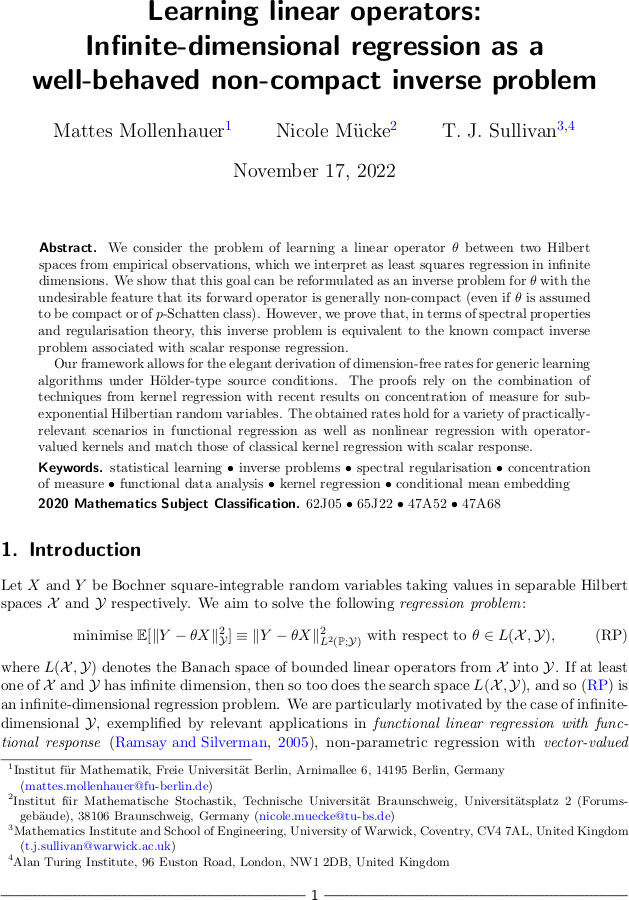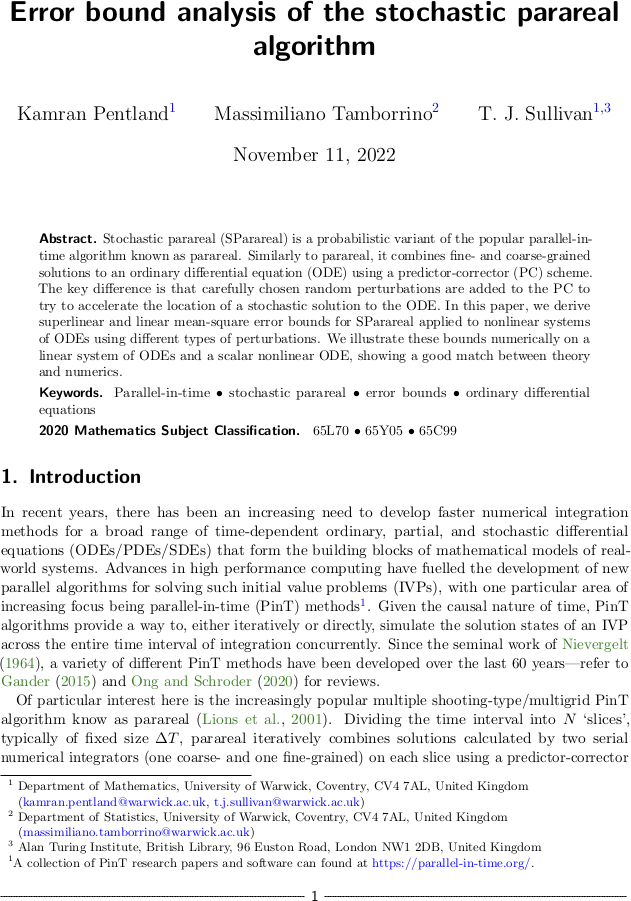# Tim Sullivan

### #preprint

Clear Search### A periodic table of modes and MAP estimators

Ilja Klebanov and I have just uploaded a preprint of our paper “A ‘periodic table’ of modes and maximum a posteriori estimators” to the arXiv.

This paper forms part of the growing body of work on the ‘small balls’ theory of modes for probability measures on metric spaces, which is needed e.g. for the treatment of MAP estimation for Bayesian inverse problems with functional unknowns. There are already several versions in the literature: the strong mode, the weak mode, and the generalised strong mode. We take an axiomatic approach to the problem and identify a system of 17 essentially distinct notions of mode, proving implications between them and providing explicit counterexamples to distinguish them. From an axiomatic point of view, all these 17 seem to be ‘equally good’, suggesting that further research is needed in this area.

Abstract. The last decade has seen many attempts to generalise the definition of modes, or MAP estimators, of a probability distribution $$\mu$$ on a space $$X$$ to the case that $$\mu$$ has no continuous Lebesgue density, and in particular to infinite-dimensional Banach and Hilbert spaces $$X$$. This paper examines the properties of and connections among these definitions. We construct a systematic taxonomy – or ‘periodic table’ – of modes that includes the established notions as well as large hitherto-unexplored classes. We establish implications between these definitions and provide counterexamples to distinguish them. We also distinguish those definitions that are merely ‘grammatically correct’ from those that are ‘meaningful’ in the sense of satisfying certain ‘common-sense’ axioms for a mode, among them the correct handling of discrete measures and those with continuous Lebesgue densities. However, despite there being 17 such ‘meaningful’ definitions of mode, we show that none of them satisfy the ‘merging property’, under which the modes of $$\mu|_{A}$$, $$\mu|_{B}$$, and $$\mu|_{A \cup B}$$ enjoy a straightforward relationship for well-separated positive-mass events $$A, B \subseteq X$$.

Published on Monday 17 July 2023 at 09:00 UTC #preprint #modes #map-estimators #klebanov### Unbounded images of Gaussian and other stochastic processes

Tadashi Matsumoto and I have just uploaded a preprint of our note “Images of Gaussian and other stochastic processes under closed, densely-defined, unbounded linear operators” to the arXiv.

The purpose of this note is to provide a self-contained rigorous proof of the well-known formula for the mean and covariance function of a stochastic process — in particular, a Gaussian process — when it is acted upon by an unbounded linear operator such as an ordinary or partial differential operator, as used in probabilistic approaches to the solution of ODEs and PDEs. This result is easy to establish in the case of a bounded operator, but the unbounded case requires a careful application of Hille's theorem for the Bochner integral of a Banach-valued random variable.

Abstract. Gaussian processes (GPs) are widely-used tools in spatial statistics and machine learning and the formulae for the mean function and covariance kernel of a GP $$v$$ that is the image of another GP $$u$$ under a linear transformation $$T$$ acting on the sample paths of $$u$$ are well known, almost to the point of being folklore. However, these formulae are often used without rigorous attention to technical details, particularly when $$T$$ is an unbounded operator such as a differential operator, which is common in several modern applications. This note provides a self-contained proof of the claimed formulae for the case of a closed, densely-defined operator $$T$$ acting on the sample paths of a square-integrable stochastic process. Our proof technique relies upon Hille's theorem for the Bochner integral of a Banach-valued random variable.

Published on Monday 8 May 2023 at 13:00 UTC #preprint #prob-num #gp #matsumoto### Learning linear operators

Mattes Mollenhauer, Nicole Mücke, and I have just uploaded a preprint of our latest article, “Learning linear operators: Infinite-dimensional regression as a well-behaved non-compact inverse problem”, to the arXiv.

Abstract. We consider the problem of learning a linear operator $$\theta$$ between two Hilbert spaces from empirical observations, which we interpret as least squares regression in infinite dimensions. We show that this goal can be reformulated as an inverse problem for $$\theta$$ with the undesirable feature that its forward operator is generally non-compact (even if $$\theta$$ is assumed to be compact or of $$p$$-Schatten class). However, we prove that, in terms of spectral properties and regularisation theory, this inverse problem is equivalent to the known compact inverse problem associated with scalar response regression. Our framework allows for the elegant derivation of dimension-free rates for generic learning algorithms under Hölder-type source conditions. The proofs rely on the combination of techniques from kernel regression with recent results on concentration of measure for sub-exponential Hilbertian random variables. The obtained rates hold for a variety of practically-relevant scenarios in functional regression as well as nonlinear regression with operator-valued kernels and match those of classical kernel regression with scalar response.

Published on Thursday 17 November 2022 at 10:00 UTC #preprint #learning #regression #mollenhauer #muecke### Error analysis for SParareal

Kamran Pentland, Massimiliano Tamborrino, and I have just uploaded a preprint of our latest article, “Error bound analysis of the stochastic parareal algorithm”, to the arXiv.

Abstract. Stochastic parareal (SParareal) is a probabilistic variant of the popular parallel-in-time algorithm known as parareal. Similarly to parareal, it combines fine- and coarse-grained solutions to an ordinary differential equation (ODE) using a predictor-corrector (PC) scheme. The key difference is that carefully chosen random perturbations are added to the PC to try to accelerate the location of a stochastic solution to the ODE. In this paper, we derive superlinear and linear mean-square error bounds for SParareal applied to nonlinear systems of ODEs using different types of perturbations. We illustrate these bounds numerically on a linear system of ODEs and a scalar nonlinear ODE, showing a good match between theory and numerics.

Published on Thursday 10 November 2022 at 10:00 UTC #preprint #prob-num #sparareal #pentland #tamborrino### Order theory and MAP estimation

Hefin Lambley and I have just uploaded a preprint of our latest article, “An order-theoretic perspective on modes and maximum a posteriori estimation in Bayesian inverse problems”, to the arXiv. On a heuristic level, modes and MAP estimators are intended to be the “most probable points” of a space $$X$$ with respect to a probability measure $$\mu$$. Thus, in some sense, they would seem to be the greatest elements of some order on $$X$$, and a rigorous order-theoretic treatment is called for, especially for cases in which $$X$$ is, say, an infinite-dimensional function space. Such an order-theoretic perspective opens up some attractive proof strategies for the existence of modes and MAP estimators but also leads to some interesting counterexamples. In particular, because the orders involved are not total, some pairs of points of $$X$$ can be incomparable (i.e. neither is more nor less likely than the other). In fact we show that there are examples for which the collection of such mutually incomparable elements is dense in $$X$$.

Abstract. It is often desirable to summarise a probability measure on a space $$X$$ in terms of a mode, or MAP estimator, i.e. a point of maximum probability. Such points can be rigorously defined using masses of metric balls in the small-radius limit. However, the theory is not entirely straightforward: the literature contains multiple notions of mode and various examples of pathological measures that have no mode in any sense. Since the masses of balls induce natural orderings on the points of $$X$$, this article aims to shed light on some of the problems in non-parametric MAP estimation by taking an order-theoretic perspective, which appears to be a new one in the inverse problems community. This point of view opens up attractive proof strategies based upon the Cantor and Kuratowski intersection theorems; it also reveals that many of the pathologies arise from the distinction between greatest and maximal elements of an order, and from the existence of incomparable elements of $$X$$, which we show can be dense in $$X$$, even for an absolutely continuous measure on $$X = \mathbb{R}$$.

Published on Monday 26 September 2022 at 09:00 UTC #preprint #modes #order-theory #map-estimators #lambley